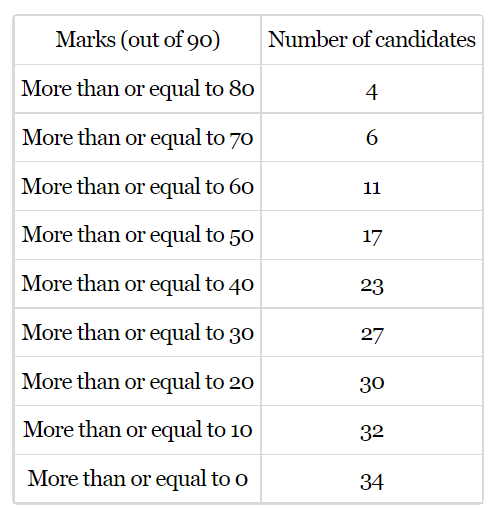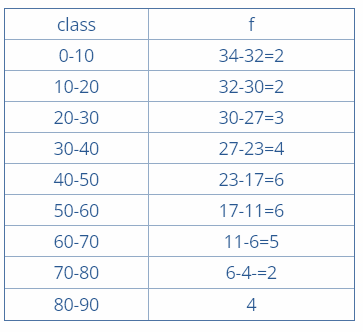State true or false.The frequency table for the data given above is:

# State true or false.The frequency table for the data given above is:1. A
True
2. B
False

Fill Out the Form for Expert Academic Guidance!l

+91

Live ClassesBooksTest SeriesSelf Learning

Verify OTP Code (required)

### Solution:

It is given that, a data of marks obtained by students.By constructing the frequency distribution table for students who have scored marks more than or equal to .
Find the frequency by subtracting the cumulative frequency from the preceding one from backwards.
Consider the class interval 80 – 90, the cumulative frequency is 4.
And so the frequency is 4.
Consider the class interval 70 -80, the cumulative frequency is 6.
And so the frequency is 6 - 4 = 2.
Consider the class interval 60 - 70, the cumulative frequency is 11.
And so the frequency is 11 - 6 = 5.
Consider the class interval 50 - 60, the cumulative frequency is 17.
And so the frequency is 17 - 11 = 6.
Consider the class interval 40 - 50, the cumulative frequency is 23.
And so the frequency is 23 - 17 = 6.
Consider the class interval 30 - 40, the cumulative frequency is 27.
And so the frequency is 27 - 23 = 4.
Consider the class interval 20 -30, the cumulative frequency is 30.
And so the frequency is 30 - 27 = 3.
Consider the class interval; 10 -20, the cumulative frequency is 32.
And so the frequency is 32 – 30 = 2.
Consider the class interval; 0 - 10, the cumulative frequency is 34.
And so the frequency is 34 - 32 = 2.
The table is given by,Hence, the given table is true.
Hence, option 1 is correct.

## Related content

 Area of Square Area of Isosceles Triangle Pythagoras Theorem Triangle Formula Perimeter of Triangle Formula Area Formulae Volume of Cone Formula Matrices and Determinants_mathematics Critical Points Solved Examples Type of relations_mathematics+91

Live ClassesBooksTest SeriesSelf Learning

Verify OTP Code (required)